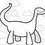# What's special about these numbers?

-1 is the only number which only 1 and itself divide it to give an integer that is not considered a prime.
1 is the multiplicative and divisive identity.
6 is the smallest perfect number.
41 is a value of n so that x^2 + x + n takes on prime values for x = 0, 1, 2, ... n-2.
53 is the only 2-digit number that is reversed is hexadecimal.
57=111 in base 7.
50 is the smallest number that can be written as the sum of 2 squares of positive numbers in 2 ways (7^2+1^2 and 5^2+5^2). 64 is the smallest number with 7 divisors.
129 is the smallest number that can be written as a sum of 3 squares of positive numbers in at least 4 ways (11^2+2^2+2^2, 10^2 + 5^2 +2^2, 8^2+8^2+1^2 and 8^2+7^2+4^2)
150 can be represented as 10010110 is base 2, 2112 in base 4 and 1100 in base 5. It also uses 2 digits an equal amount of times.Note by Kenny O.
3 years, 11 months ago

This discussion board is a place to discuss our Daily Challenges and the math and science related to those challenges. Explanations are more than just a solution — they should explain the steps and thinking strategies that you used to obtain the solution. Comments should further the discussion of math and science.

When posting on Brilliant:

• Use the emojis to react to an explanation, whether you're congratulating a job well done , or just really confused .
• Ask specific questions about the challenge or the steps in somebody's explanation. Well-posed questions can add a lot to the discussion, but posting "I don't understand!" doesn't help anyone.
• Try to contribute something new to the discussion, whether it is an extension, generalization or other idea related to the challenge.

MarkdownAppears as
*italics* or _italics_ italics
**bold** or __bold__ bold
- bulleted- list
• bulleted
• list
1. numbered2. list
1. numbered
2. list
Note: you must add a full line of space before and after lists for them to show up correctly
paragraph 1paragraph 2

paragraph 1

paragraph 2

[example link](https://brilliant.org)example link
> This is a quote
This is a quote
    # I indented these lines
# 4 spaces, and now they show
# up as a code block.

print "hello world"
# I indented these lines
# 4 spaces, and now they show
# up as a code block.

print "hello world"
MathAppears as
Remember to wrap math in $$ ... $$ or $ ... $ to ensure proper formatting.
2 \times 3 $2 \times 3$
2^{34} $2^{34}$
a_{i-1} $a_{i-1}$
\frac{2}{3} $\frac{2}{3}$
\sqrt{2} $\sqrt{2}$
\sum_{i=1}^3 $\sum_{i=1}^3$
\sin \theta $\sin \theta$
\boxed{123} $\boxed{123}$

Sort by:

I suggest you to use $Latex$ for bringing more effectiveness .

- 3 years ago